# Bouwer and Rice Slug Test Solution for Unconfined Aquifers

Related Solution Methods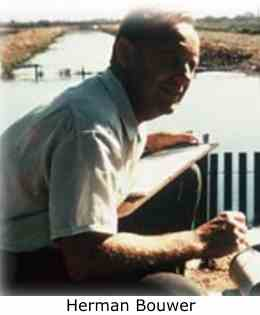A mathematical solution by Bouwer and Rice (1976) is useful for determining hydraulic conductivity ($K$) of an unconfined aquifer from an overdamped slug test. Analysis involves matching a straight line to water-level displacement data collected over time.

Herman Bouwer (1927-2013) and Bob Rice used a resistance-network analog to develop an empirical model for rate of water-level recovery during a slug test in an unconfined aquifer. Their solution places a constant-head boundary at the water table and the control well may be fully or partially penetrating. Like the Hvorslev method, the Bouwer and Rice model assumes quasi-steady state flow by neglecting aquifer storativity. Subsequent modifications to the solution account for filter pack drainage (Bouwer 1989) and hydraulic conductivity anisotropy (Zlotnik 1994).

where

• $H$ is displacement at time $t$ [L]
• ${H}_{0}$ is initial displacement at $t=0$ [L]
• ${K}_{\text{r}}$ is radial (horizontal) hydraulic conductivity [L/T]
• ${K}_{\text{z}}$ is vertical hydraulic conductivity [L/T]
• $L$ is screen length [L]
• ${n}_{\text{e}}$ is effective porosity (specific yield) of the filter pack [dimensionless]
• ${r}_{\text{c}}$ is nominal casing radius [L]
• ${r}_{\text{w}}$ is well radius [L]
• ${R}_{\text{e}}$ is external or effective radius of the test [L]
• $t$ is elapsed time since initiation of the test [T]

The term $\mathrm{ln}\left({R}_{\text{e}}/{r}_{\mathrm{we}}\right)$ in Equation 1 is an empirical quantity determined from the analog model that accounts for well-aquifer geometry.

Note that ${r}_{\text{w}}$ is typically taken as the borehole radius (i.e., extending to the outer radius of the filter pack) when the filter pack is expected to be more conductive than the aquifer.

AQTESOLV allows you to use the effective casing radius in Equation 2 when response data from a well screened across the water table exhibit a double-straight line appearance due to filter pack drainage. No correction to the casing radius is necessary when the screen is fully submerged below the water table or the aquifer is confined.

also includes recommended head ranges (Butler 1998) for the Bouwer and Rice solution that can reduce ambiguity when interpreting response data with a concave upward appearance.

Although developed originally for unconfined aquifers, Bouwer (1989) noted the utility of the Bouwer and Rice method for slug tests in confined aquifers as well.

## Assumptions

The following assumptions apply to the use of the Bouwer and Rice slug test method:

• aquifer has infinite areal extent
• aquifer is homogeneous and of uniform thickness
• aquifer potentiometric surface is initially horizontal
• control well is fully or partially penetrating
• a volume of water, V, is injected or discharged instantaneously from the control well
• aquifer is confined or unconfined
• flow is steady

## SolutionOptions

provides the following options for the Bouwer and Rice method for overdamped slug tests:

• partially penetrating wells
• correction for gravel pack porosity
• hydraulic conductivity anisotropy

## Benchmarks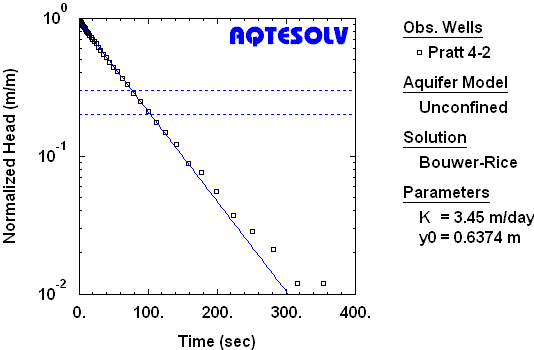AQTESOLV benchmark for Bouwer and Rice (1976) solution matched to an overdamped slug test in an unconfined aquifer using recommended head range (Butler 1998). Butler (1998) reported K=3.46 m/day for these data.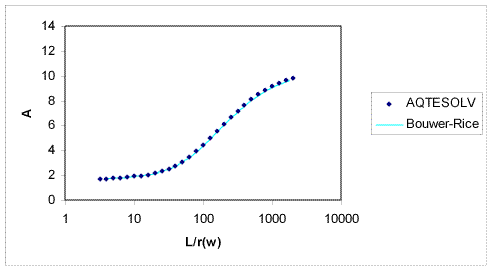Benchmark graph comparing "A" coefficient curve (line) from Bouwer and Rice (1976, Figure 3) and values computed by AQTESOLV (symbols).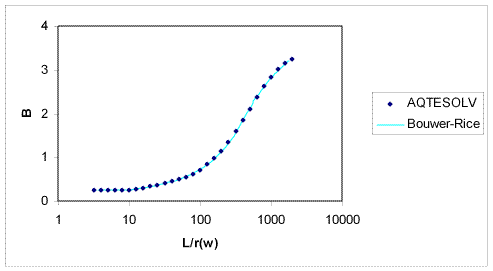Benchmark graph comparing "B" coefficient curve (line) from Bouwer and Rice (1976, Figure 3) and values computed by AQTESOLV (symbols).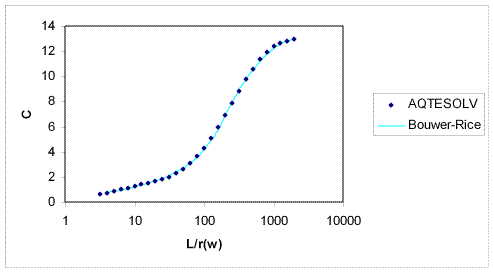Benchmark graph comparing "C" coefficient curve (line) from Bouwer and Rice (1976, Figure 3) and values computed by AQTESOLV (symbols).